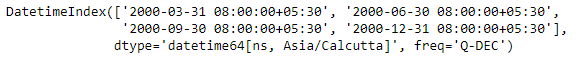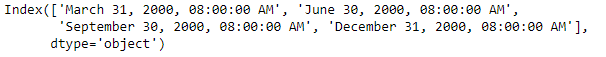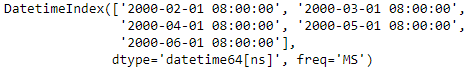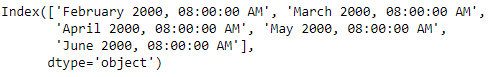Skip to content
Related Articles
Python | Pandas DatetimeIndex.strftime()
• Last Updated : 24 Dec, 2018

Python is a great language for doing data analysis, primarily because of the fantastic ecosystem of data-centric python packages. Pandas is one of those packages and makes importing and analyzing data much easier.

Pandas` DatetimeIndex.strftime()` function convert to Index using specified date_format. The function return an Index of formatted strings specified by date_format, which supports the same string format as the python standard library.

Syntax: DatetimeIndex.strftime(date_format)

Parameters :
date_format : Date format string (e.g. “%Y-%m-%d”).

Return : Index of formatted strings

Example #1: Use `DatetimeIndex.strftime()` function to convert the given DatetimeIndex object to the specified format.

 `# importing pandas as pd``import` `pandas as pd`` ` `# Create the DatetimeIndex``# Here 'Q' represents quarter end frequency ``didx ``=` `pd.DatetimeIndex(start ``=``'2000-01-15 08:00'``, freq ``=``'Q'``,``                            ``periods ``=` `4``, tz ``=``'Asia/Calcutta'``)`` ` `# Print the DatetimeIndex``print``(didx)`

Output :Now we want to convert the given DatetimeIndex object to `('%B %d, %Y, %r')` format.

 `# change the datetime format.``didx.strftime(``'% B % d, % Y, % r'``)`

Output :As we can see in the output, the function has changed the format of the DatetimeIndex object to the desired format.

Example #2: Use `DatetimeIndex.strftime()` function to convert the given DatetimeIndex object to the specified format.

 `# importing pandas as pd``import` `pandas as pd`` ` `# Create the DatetimeIndex``# Here 'MS' represents month start frequency ``didx ``=` `pd.date_range(pd.Timestamp(``"2000-01-15 08:00"``),``                              ``periods ``=` `5``, freq ``=``'MS'``)`` ` `# Print the DatetimeIndex``print``(didx)`

Output :Now we want to convert the given DatetimeIndex object to `('%B %Y, %r')` format.

 `# change the datetime format.``didx.strftime(``'% B % Y, % r'``)`

Output :As we can see in the output, the function has changed the format of the DatetimeIndex object to the desired format.

Attention geek! Strengthen your foundations with the Python Programming Foundation Course and learn the basics.

To begin with, your interview preparations Enhance your Data Structures concepts with the Python DS Course. And to begin with your Machine Learning Journey, join the Machine Learning – Basic Level Course

My Personal Notes arrow_drop_up